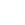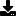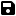#### Browse / Search / Login# Base Changing

by Infra (7 Submissions)
Category: Encryption
Compatability: Visual Basic 3.0
Difficulty: Intermediate
Date Added: Wed 3rd February 2021
Rating:(17 Votes)

Lately, I've seen a couple binary convertion functions. I decided to "up" how powerful the converters are. I've created a base convertion that can convert 2 (2 is used to create binary) to 9. There is also a converter to convert everything back to normal. So, let's say you want to convert 150 to binary, and put it in the string Binary:
Binary\$ = Base(2, 150, True)
And if you want to convert it back:
Binary\$ = Dec(2, Binary\$)
Simple as that. You can also convert to other bases, which could be useful in an encryption (if you really want to confuse crackers). There are also comments on virtually EVERY line. All in all, this is a must see!

Inputs
BaseNum needs to be an integer, from 2 to 9 (program will filter out any other numbers).

Rate Base Changing

`'If you want to use this in a program, e-mail me for permission... Howabout you just e-mail me the program when you're done so I can mess with it instead. That's the only reason I have that permission thing anyways.Function Base(BaseNum As Integer, Number As Integer, ClipZeros As Boolean) As StringDim i As Integer, MB As Integer, endstr As StringIf BaseNum > 9 Or BaseNum < 2 Then Exit Function 'Filter out "bad" numbersMB = MaxBit(BaseNum) 'Get the maximum amount of bits possibleendstr\$ = "" 'I know, this isn't needed... But it makes me feel secure :)If MB = 0 Then Exit Function 'This also makes me feel secureFor i = 1 To MB 'You know this If BaseNum ^ (MB - i) <= Number Then 'If I can get one of the BaseNum ^ (MB - i)'s out of Number endstr\$ = endstr\$ & Int(Number / (BaseNum ^ (MB - i))) 'This will see how many BaseNum things are in Number, and put them in as a digit on the end string Number = Number - (Int(Number / (BaseNum ^ (MB - i))) * (BaseNum ^ (MB - i))) 'This will subtract everything that was put in the end string Else 'This is if Number fails its test endstr\$ = endstr\$ & "0" 'Add a 0, needed if you are going to have accuracy in here End If 'Comments on every line, live with itNext i 'Loop the iIf ClipZeros = True Then 'If we need to clip off the 0's at the start Do While Mid\$(endstr\$, 1, 1) = "0" 'When there is a zero in front... endstr\$ = Mid\$(endstr\$, 2, Len(endstr\$) - 1) 'Take it off... Loop 'And check againEnd If 'I don't know what to put here, sorryBase = endstr\$ 'Return the number string to the functionEnd Function 'End the function, what else?Function Dec(OldBaseNum As Integer, Number As String) As IntegerDim i As Integer, MB As Integer, endstr As StringIf OldBaseNum > 9 Or OldBaseNum < 2 Then Exit Function 'Make sure the numbers are in the right areaMB = MaxBit(OldBaseNum) 'Get the maximum possible bits without blowing up vbDo While Len(Number) < MB 'As long as the number doesn't have all of the extra 0's...Number = "0" & Number 'Add another...Loop 'And check againFor i = 1 To MB 'What am I supposed to put? Sorry, I'll be serious now, just bored.endstr = Val(endstr\$) + (OldBaseNum ^ (MB - i) * Mid(Number, i, 1)) 'This will see how much each bit is worth, and multiply it by the actual value of itNext i 'BleahDec = Val(endstr) 'This will return the number to the functionEnd Function 'End the functionFunction MaxBit(BaseNum As Integer)Dim i As Integer, MB As Integer, buffer As IntegerOn Error GoTo GotNum 'This is needed, you'll see whyMB = 0 'I like to do thatFor i = 1 To 20 'Start the i "loop"buffer = BaseNum ^ i 'Buffer isn't used, I'll explain why. Vb will give an error when it reaches above the integer limit with that exponent. Everytime it makes it, it adds to the exponent, eventually making it to the max number of bits that can be in the number string. Get it? If you don't, look at the Base function and this function VERY carefully.MB = MB + 1 'This adds to the exponentNext i 'Loops the iGotNum: 'This is where it goes when it reaches the max bits possibleMaxBit = MB 'This will just return the value to the function, and send it over to the other 2End Function 'End the function, duh`Download this snippetAdd to My Saved Code